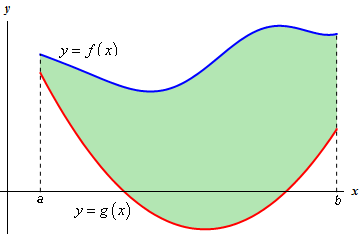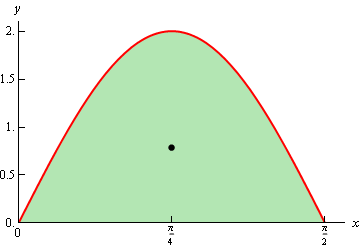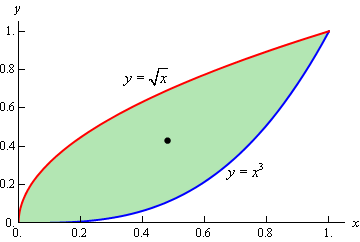Paul's Online Notes
Home / Calculus II / Applications of Integrals / Center of Mass
Show Mobile Notice Show All Notes Hide All Notes
Mobile Notice
You appear to be on a device with a "narrow" screen width (i.e. you are probably on a mobile phone). Due to the nature of the mathematics on this site it is best views in landscape mode. If your device is not in landscape mode many of the equations will run off the side of your device (should be able to scroll to see them) and some of the menu items will be cut off due to the narrow screen width.

### Section 8.3 : Center Of Mass

In this section we are going to find the center of mass or centroid of a thin plate with uniform density $$\rho$$. The center of mass or centroid of a region is the point in which the region will be perfectly balanced horizontally if suspended from that point.

So, let’s suppose that the plate is the region bounded by the two curves $$f\left( x \right)$$ and $$g\left( x \right)$$ on the interval $$\left[ {a,b} \right]$$. So, we want to find the center of mass of the region below.We’ll first need the mass of this plate. The mass is,

\begin{align*}M & = \rho \left( {{\mbox{Area of plate}}} \right)\\ & = \rho \int_{{\,a}}^{{\,b}}{{f\left( x \right) - g\left( x \right)\,dx}}\end{align*}

Next, we’ll need the moments of the region. There are two moments, denoted by $${M_x}$$ and $${M_y}$$. The moments measure the tendency of the region to rotate about the $$x$$ and $$y$$-axis respectively. The moments are given by,

#### Equations of Moments

\begin{align*}{M_x} & = \rho \int_{{\,a}}^{{\,b}}{{\frac{1}{2}\left( {{{\left[ {f\left( x \right)} \right]}^2} - {{\left[ {g\left( x \right)} \right]}^2}} \right)\,dx}}\\ {M_y} & = \rho \int_{{\,a}}^{{\,b}}{{x\left( {f\left( x \right) - g\left( x \right)} \right)\,dx}}\end{align*}

The coordinates of the center of mass, $$\left( {\overline{x},\overline{y}} \right)$$, are then,

#### Center of Mass Coordinates

\begin{align*}\overline{x} & = \frac{{{M_y}}}{M} = \frac{{\int_{{\,a}}^{{\,b}}{{x\left( {f\left( x \right) - g\left( x \right)} \right)\,dx}}}}{{\int_{{\,a}}^{{\,b}}{{f\left( x \right) - g\left( x \right)\,dx}}}} = \frac{1}{A}\int_{{\,a}}^{{\,b}}{{x\left( {f\left( x \right) - g\left( x \right)} \right)\,dx}}\\ \overline{y} & = \frac{{{M_x}}}{M} = \frac{{\int_{{\,a}}^{{\,b}}{{\frac{1}{2}\left( {{{\left[ {f\left( x \right)} \right]}^2} - {{\left[ {g\left( x \right)} \right]}^2}} \right)\,dx}}}}{{\int_{{\,a}}^{{\,b}}{{f\left( x \right) - g\left( x \right)\,dx}}}} = \frac{1}{A}\int_{{\,a}}^{{\,b}}{{\frac{1}{2}\left( {{{\left[ {f\left( x \right)} \right]}^2} - {{\left[ {g\left( x \right)} \right]}^2}} \right)\,dx}}\end{align*}

where,

$A = \int_{{\,a}}^{{\,b}}{{f\left( x \right) - g\left( x \right)\,dx}}$

Note that the density, $$\rho$$, of the plate cancels out and so isn’t really needed.

Let’s work a couple of examples.

Example 1 Determine the center of mass for the region bounded by $$y = 2\sin \left( {2x} \right)$$, $$y = 0$$ on the interval $$\left[ {0,\displaystyle \frac{\pi }{2}} \right]$$.
Show Solution

Here is a sketch of the region with the center of mass denoted with a dot.Let’s first get the area of the region.

\begin{align*}A & = \int_{{\,0}}^{{\,\frac{\pi }{2}}}{{2\sin \left( {2x} \right)\,dx}}\\ & = \left. { - \cos \left( {2x} \right)} \right|_0^{\frac{\pi }{2}}\\ & = 2\end{align*}

Now, the moments (without density since it will just drop out) are,

\begin{array}{*{20}{c}}\begin{aligned}{M_x} & = \int_{{\,0}}^{{\,\frac{\pi }{2}}}{{2{{\sin }^2}\left( {2x} \right)\,dx}}\\ & = \int_{{\,0}}^{{\,\frac{\pi }{2}}}{{1 - \cos \left( {4x} \right)\,dx}}\\ & = \left. {\left( {x - \frac{1}{4}\sin \left( {4x} \right)} \right)} \right|_0^{\frac{\pi }{2}}\\ & = \frac{\pi }{2}\end{aligned}& \hspace{0.5in} &\begin{aligned}{M_y} & = \int_{{\,0}}^{{\,\frac{\pi }{2}}}{{2x\sin \left( {2x} \right)\,dx}}\hspace{0.25in}{\mbox{integrating by parts}}...\\ & = - \left. {x\cos \left( {2x} \right)} \right|_0^{\frac{\pi }{2}} + \int_{{\,0}}^{{\,\frac{\pi }{2}}}{{\cos \left( {2x} \right)\,dx}}\\ & = - \left. {x\cos \left( {2x} \right)} \right|_0^{\frac{\pi }{2}} + \left. {\frac{1}{2}\sin \left( {2x} \right)} \right|_0^{\frac{\pi }{2}}\\ & = \frac{\pi }{2}\end{aligned}\end{array}

The coordinates of the center of mass are then,

\begin{align*}\overline{x} & = \frac{{{}^{\pi }/{}_{2}}}{2} = \frac{\pi }{4}\\ \overline{y} & = \frac{{{}^{\pi }/{}_{2}}}{2} = \frac{\pi }{4}\end{align*}

Again, note that we didn’t put in the density since it will cancel out.

So, the center of mass for this region is $$\left( {\frac{\pi }{4},\frac{\pi }{4}} \right)$$.

Example 2 Determine the center of mass for the region bounded by $$y = {x^3}$$ and $$y = \sqrt x$$.
Show Solution

The two curves intersect at $$x = 0$$ and $$x = 1$$ and here is a sketch of the region with the center of mass marked with a box.We’ll first get the area of the region.

\begin{align*}A & = \int_{{\,0}}^{{\,1}}{{\sqrt x - {x^3}\,dx}}\\ & = \left. {\left( {\frac{2}{3}{x^{\frac{3}{2}}} - \frac{1}{4}{x^4}} \right)} \right|_0^1\\ & = \frac{5}{{12}}\end{align*}

Now the moments, again without density, are

\begin{array}{*{20}{c}}\begin{aligned}{M_x} & = \int_{{\,0}}^{{\,1}}{{\frac{1}{2}\left( {x - {x^6}} \right)\,dx}}\\ & = \left. {\frac{1}{2}\left( {\frac{1}{2}{x^2} - \frac{1}{7}{x^7}} \right)} \right|_0^1\\ & = \frac{5}{{28}} \\ & \end{aligned}& \hspace{0.5in} &\begin{aligned}{M_y} & = \int_{{\,0}}^{{\,1}}{{x\left( {\sqrt x - {x^3}} \right)\,dx}}\\ & = \int_{{\,0}}^{{\,1}}{{{x^{\frac{3}{2}}} - {x^4}\,dx}}\\ & = \left. {\left( {\frac{2}{5}{x^{\frac{5}{2}}} - \frac{1}{5}{x^5}} \right)} \right|_0^1\\ & = \frac{1}{5}\end{aligned}\end{array}

The coordinates of the center of mass is then,

\begin{align*}\overline{x} & = \frac{{{1}/{5}\;}}{{{5}/{{12}}\;}} = \frac{{12}}{{25}}\\ \overline{y} & = \frac{{{5}/{{28}}\;}}{{{5}/{{12}}\;}} = \frac{3}{7}\end{align*}

The coordinates of the center of mass are then,$$\left( {\frac{{12}}{{25}},\frac{3}{7}} \right)$$.